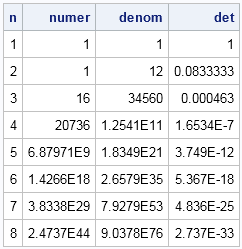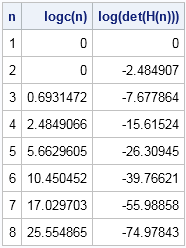Last week I described the Hilbert matrix of size n, which is a famous square matrix in numerical linear algebra. It is famous partially because its inverse and its determinant have explicit formulas (that is, we know them exactly), but mainly because the matrix is ill-conditioned for moderate values of n. Consequently, a small Hilbert matrix (often n < 10) can be used to test numerical algorithms to see how they perform on an ill-conditioned or nearly singular matrix.

The Hilbert matrix can teach us a lesson about how to compute with large numbers. The determinant of the Hilbert matrix has an explicit formula that involves the product of factorials. If you define the function
c(n) = 1! · 2! · ... · (n – 1)!
then the determinant of the Hilbert matrix of size n is
det(Hn) = c(n)4 / c(2n)
This ratio goes to zero very quickly as n increases. Yes, the numerator is large, but the denominator is even larger, as shown by the following SAS/IML program:

```proc iml; start c(n); return( prod(fact(1:n-1)) ); /* 1!*2!*...*(n-1)! */ finish;   maxN = 8; numer = j(maxN,1); denom = j(maxN,1); det = j(maxN,1); do n = 1 to maxN; numer[n] = c(n)##4; denom[n] = c(2*n); det[n] = numer[n] / denom[n]; end; print (T(1:maxN))[L="n"] numer denom det;```You can see that the determinant of the Hilbert matrix is practically zero for n > 5, so most numerical algorithms would conclude that the matrix is singular. However, in exact arithmetic, the determinant of the Hilbert matrix is the reciprocal of a large integer, so it is always positive.

### How to numerically compute with super-factorial quantities?

The Hilbert matrix illustrates two common problems that arise when computing with huge numbers. First, the function c(n) results in a numerical overflow when n > 27, so we cannot use that function to compute the determinant for more than a 13 x 13 Hilbert matrix. Second, the determinant computation involves the ratio of huge numbers, so the accuracy of the determinant is questionable.

A standard technique for computing with such huge numbers is to transform the quantities, usually by taking logarithms. If we can compute the log-determinant, then we "know" the value of the actual determinant even when we cannot compute the determinant with double-precision arithmetic.

I have previously shown how to numerically compute the log-determinant of a general matrix. However, for the Hilbert matrix the determinant is known exactly, so we can compute the exact log-determinant. The formula for the log-determinant is log(det(Hn)) = 4*log(c(n)) – log(c(2n)).

Although c(n) involves products of factorials, log(c(n)) only involves the sums of logs of integers. Why is that? Well, since the log of a product is the sum of the logs, the quantity log(k!) can be computed in the SAS/IML language as sum(log(1:k)). Likewise, the product of factorials reduces to a sum of log-factorials, as shown in the following SAS/IML function for the quantity log(c(n)):

```/* compute log(c(n)) */ start logc(n); s = 0; /* log(1!) */ do i = 2 to n-1; s = s + sum(log(1:i)); end; return( s ); finish;   /* compute log(c(n)) and the log-determinant of the Hilbert matrix */ logcn = j(maxN,1); logdet = j(maxN,1); do n = 1 to maxN; logcn[n] = logc(n); logdet[n] = 4*logc(n) - logc(2*n); end; print (T(1:maxN))[L="n"] logcn[L="logc(n)"] logdet[L="log(det(H(n)))"];```The difference is amazing. Whereas the quantity c(8) is huge and the quantity det(H8)) is tiny, the logs of these quantities are reasonable sizes. Although c(100) is astronomically huge, you can easily compute the log of c(100) and the log of det(H100)).

You might never have a need to compute the determinant of a Hilbert matrix, but the ideas in this article apply to many similar computational situations. For example, in maximum likelihood estimate, statistical software usually computes the log-likelihood function rather than the likelihood function. So next time that you find yourself computing a quantity that involve huge numbers (or tiny numbers), consider computing those quantities on a logarithmic scale. It will make a huge difference.

Share1.